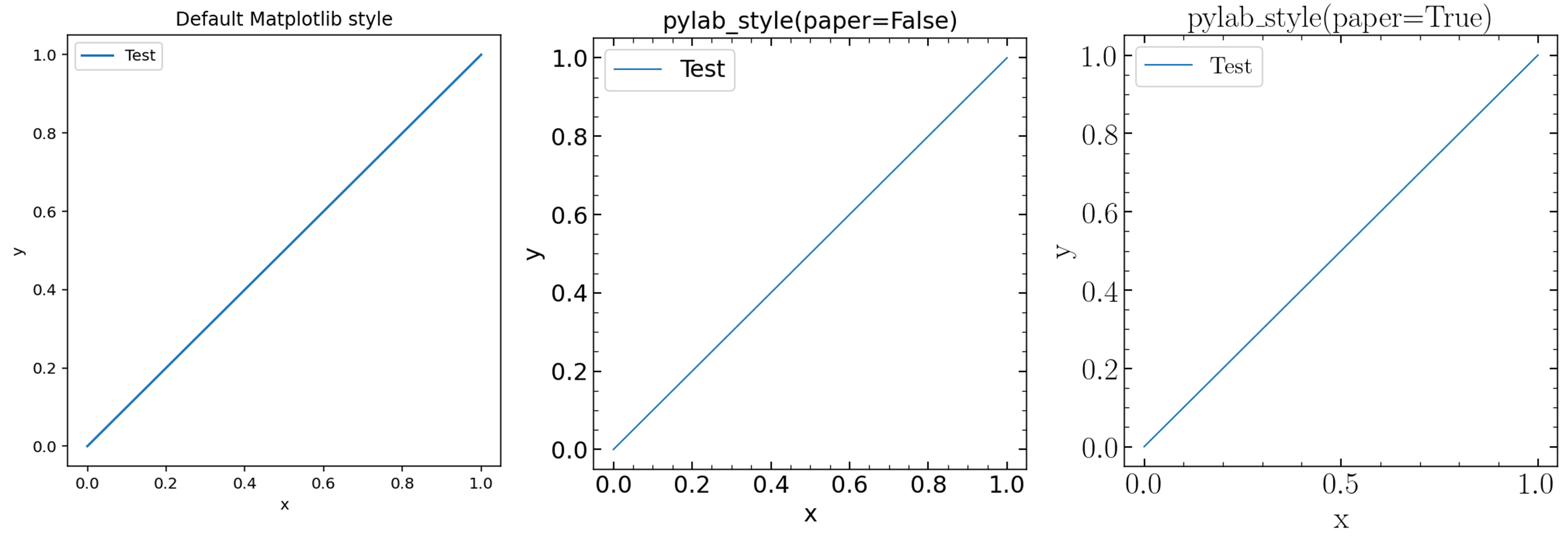# Publications using astroNN

1. Deep learning of multi-element abundances from high-resolution spectroscopic data
Henry W. Leung, Jo Bovy (2019)
Original astroNN paper
2. Dynamical heating across the Milky Way disc using APOGEE and Gaia
J. Ted Mackereth, Jo Bovy, Henry W. Leung, et al. (2019)
Use ApogeeBCNN to infer spectroscopic age
3. Simultaneous calibration of spectro-photometric distances and the Gaia DR2 parallax zero-point offset with deep learning
Henry W. Leung, Jo Bovy (2019)
Use ApogeeDR14GaiaDR2BCNN to infer spectro-photometric distances
4. Solar image denoising with convolutional neural networks
C. J. Díaz Baso, J. de la Cruz Rodríguez, S. Danilovic (2019)
5. A variational encoder-decoder approach to precise spectroscopic age estimation for large Galactic surveys
Henry W. Leung, Jo Bovy, J. Ted Mackereth, Andrea Miglio (2023)
Use ApokascEncoderDecoder to infer spectroscopic age trained on APOGEE and Kepler

## Publication figure style

astroNN contains a function that helps me to standardize maplotlib figure style used in my publication.

astroNN.shared.matplotlib.pylab_style(paper=False)[source]

Such function can be used by simply calling it before using matplotlib to plot any figure

1import matplotlib.pylab as plt
2from astroNN.shared import pylab_style
3
4pylab_style(paper=True)
5
6# matplotlib code goes here


If you do not have $$\LaTeX$$ installed on your computer, you can set the paper option to False like pylab_style(paper=False)

Here are a figure that compare different styles using the following matplotlib code

1plt.figure(figsize=(5, 5))
2plt.plot([0, 1], [0, 1], label="Test")
3plt.xlabel("x")
4plt.ylabel("y")
5plt.legend()